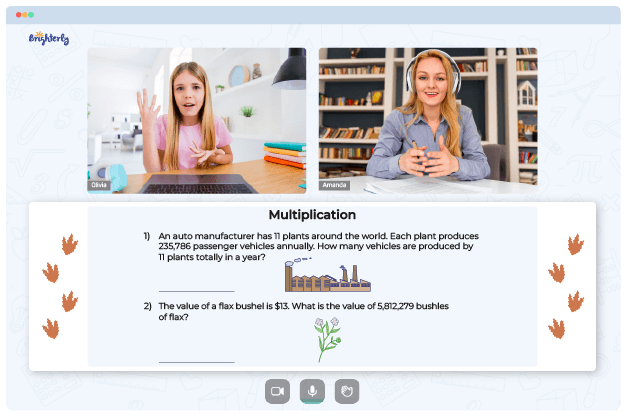# Multiplication Worksheets for 5th Graders

Multiplication is simple, but you need constant practice to perfect it. With multiplication worksheets for 5th grade, children can learn math unaided. An excellent 5th grade math multiplication worksheet will let you practice with your child at home. Proceed reading to learn which worksheets we recommend and where you can find them.

Before we start

Multiplication is one of the critical concepts in basic math since your kid will keep dealing with it in more complex forms as they move from grade to grade and even after high school. Therefore, a solid foundation in multiplication is a must have for every child.

To understand multiplication in its simple and complex forms, your child should first learn the principles of multiplication. We recommend booking a free demo with Brighterly to see how well your child can navigate these principles.

## Free 5th Grade Multiplication Worksheets from Brighterly’s Tutors

Brighterly’s tutors understand that teaching your child math at home can be tiring, especially without a strategy and professional help. And with the newly implemented common core math, parents need more than pre-existing math knowledge to help their children learn. Here are some types of free 5th grade multiplication worksheets that you can use:

Some kids find the idea of equal groups confusing in multiplication. So, it is important to simplify it for children using visual techniques. Consider using a 5th grade multiplication worksheet that uses illustrations to explain equal groups and limits students’ math problems to multiplications of numbers between 1 – 10.

### Multiply single-digit numbers by multiples of 10

During multiplication practice, 5th grade students often struggle with large numbers. Using multiplication worksheets, 5th grade kids can learn to multiply double-digit numbers by single-digit ones. Many multiplication worksheets teach 5th graders to multiply by tens, with equations like 5 x 10, 4 x 10, 9 x 10, etc.

### Multiplication sentences

The multiplication sentence concept frames multiplication tasks as word problems to add an extra layer of difficulty to the challenge. With 5th grade math worksheets, multiplication sentences become easy to break down. These worksheets help students separate numbers from sentences to understand them as separate variables.

## Printable PDFs of Grade 5 Multiplication Worksheets

You can download printable multiplication worksheets for 5th graders in PDF format. They are available online, easy to understand, and are perfect teaching aids for math tutors. With printable multiplication worksheets, grade 5 students can practice math even after school.### Multiply Fractions### Multiply Three or More Numbers Up to 100### Multiply Three Numbers Up to 1000## Good Times Await with Multiplication Worksheets for Grade 5

As a parent or a teacher, you no longer have to go through rigorous teaching processes to help your child understand multiplication. The entire ordeal is easier with worksheets. These materials contain images and patterns to guide 5th graders through multiplication problems and help them become more confident in their math skills.

This 5th grade multiplication worksheet is an excellent resource for students to practice their multiplication skills. It is designed to help the student understand the concepts of multiplication and develop a strong foundation in this mathematics skill. The worksheet focuses on single-digit, double-digit, and triple-digit multiplication problems with various difficulty levels. Visual aids are provided to help the student better understand the concepts and practice applying them.

The worksheet also includes a comprehensive answer key, so parents and teachers can easily check the students’ progress. Additionally, review questions are provided at the end of each section to ensure that the student has full understanding of all topics covered in the worksheet. With its varied difficulty levels, this 5th grade multiplication worksheet is an excellent resource to help students develop strong multiplication skills. It is sure to help the student succeed in class and on tests. Get your copy today!

### More Multiplication Worksheets

Problems with Multiplication?• Start learning with an online tutor.

Is your child finding it challenging to master the fundamentals of multiplication? An online tutor could be the solution.